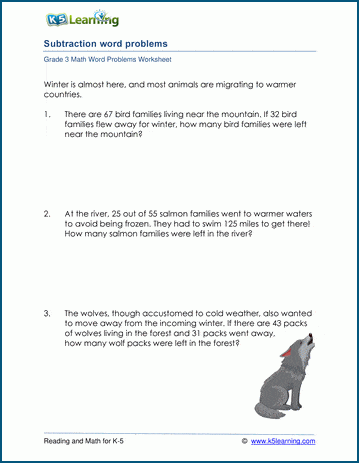# Subtraction Word Problem Worksheets for 3rd Grade

## Simple Subtraction Word Problems

These word problems are at a grade 3 level and use simple (horizontal) subtraction.  The student should read the problem, write a subtraction equation from it and then solve the problem using mental subtraction.  Students should have a good understanding of the meaning of subtraction and have studied their subtraction math facts prior to attempting these worksheets.

## Subtraction in Columns Word Problems

The student will derive a subtraction equation from the math word problem, then use column form subtraction to solve it.  Some problems may require borrowing across columns.  Most numbers are 2 or 3 digits.  Students should be comfortable with subtraction in columns before attempting these worksheets.

After the student has mastered these worksheets, they may want to try our mixed addition and subtraction world problem worksheets.  Mixed worksheets enhance the challenge for the student.Sample Grade 3 Subtraction Word Problem Worksheet

## More word problem worksheets

Explore all of our math word problem worksheets, from kindergarten through grade 5.

## What is K5?

K5 Learning offers reading and math worksheets, workbooks and an online reading and math program for kids in kindergarten to grade 5.  We help your children build good study habits and excel in school.